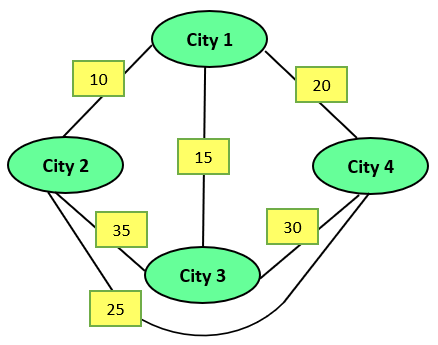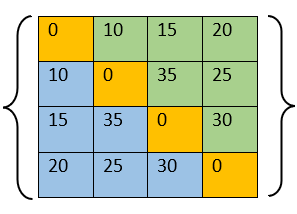# Travelling Salesman Problem | Part 2

## Introduction

The travelling salesman problem is one of the most searched optimisation problems. Many optimisation methods use the travelling salesman problem as a benchmark. Despite the problem's computational difficulty, various algorithms exist. These algorithms allow instances with tens of thousands of cities to be solved completely.

In this article, we’ll be looking at the dynamic programming approach for the travelling salesman problem.

So, Let’s get started!

## Problem Statement

The problem states that "What is the shortest possible route that helps the salesman visits each city precisely once and returns to the origin city, provided the distances between each pair of cities given?"

Let’s understand this with the help of an example.

Given, City1, City2, City3, City4 and distances between them as shown below. A salesman has to start from City 1, travel through all the cities and return to City1. There are various possible paths, but the problem is to find the shortest possible route.

The various possible routes are:

Since we are travelling in a loop(source and destination are the same), paths like (1 - 2 - 3 - 4 - 1) and (1 - 4 - 3 - 2 - 1) are considered identical.

Out of the above three routes, route 2 is optimal. So, this will be the answer.

Let’s understand the solution approach to this problem.

## Solution Approach(Dynamic programming)

A dynamic programming paradigm is used when we have problems that can be decomposed into similar sub-problems. In this paradigm, the results of the subproblems are stored to reuse later. These algorithms are primarily used for optimisation.

As we saw earlier, TSP is an optimisation problem. To apply the DP technique, we need to find out if TSP can be divided into similar subproblems or not.

Let's take the above example. The graph and its cost adjacency matrix is given below:We can observe that the adjacency cost matrix is symmetric. By symmetric, we mean the distance between cities 2 to 3 is the same as cities 3 to 2.

Let’s take a tour T from city 1 to any of the cities 2, 3, 4 as T (1,{2,3,4}). There are three possible ways to complete this tour.

Case 1: If he visits from City 1 to 2, this further depends on a subproblem, i.e. visiting from City 2 to City 3 or 4.

Case 2: If he visits from City 1 to 3, this further depends on a subproblem, i.e. visiting from City 3 to City 2 or 4.

Case 3: If he visits from City 1 to 4, this further depends on a subproblem, i.e. visiting from City 4 to City 2 or 3.

Here, we can observe that TSP can be divided into subproblems. This means we can use dynamic programming techniques to solve this problem.

Now, let’s see the dynamic programming approach in detail of the tour mentioned above:

Let the equation 1 be:

The above problem depends on some new subproblems. Let’s solve them first.

Now putting the values of these subproblems in equation 1

Here, the tour having the path 1 -> 2 -> 4 -> 3 -> 1 and 1 -> 3 -> 4 -> 2 -> 1 are same and having the minimum cost as 80.

## Implementation

Here, we are using the DP array to store the results of the subproblems. We are using bit masking techniques to keep track of the cities that are visited.

Output

### Time and Space Complexity

• The time complexity is O (n22n), where n is the number of cities.

Reason: While solving recursive equations, we will get a total (n-1) 2(n-2)  sub-problems, which is O (n2n). Each sub-problem will take O (n) time to find a path to remaining (n-1) cities.

Therefore total time complexity is O (n2n)O (n)O (n22n)

• Space complexity is O (n2n).

The implementation of the travelling salesman problem using the brute force approach is explained in Part-1. So, go check it out!

1. What is TSP?
Answer: TSP is the travelling salesman problem consists of a salesperson and his travel to various cities. The salesperson must travel to each of the cities, beginning and ending in the same.

2. What type of problem is TSP?
Answer: TSP is an optimisation problem. In this, we have to optimise the route of travel.

3. How is TSP related to the minimum spanning tree?
Answer: TSP and MST are two algorithmic problems that are closely connected. In particular, an open-loop TSP solution is a spanning tree, although it is not always the shortest spanning tree.

## Key Takeaways

In this article, we ran you through the travelling salesman problem. We saw a dynamic programming approach to solve the problem.

There are various algorithmic paradigms such as Dynamic Programming and Backtracking to solve this problem. We saw earlier that TSP is somewhat related to the minimum spanning tree. You can visit these two outstanding articles on the Prims and Kruskal's algorithm.